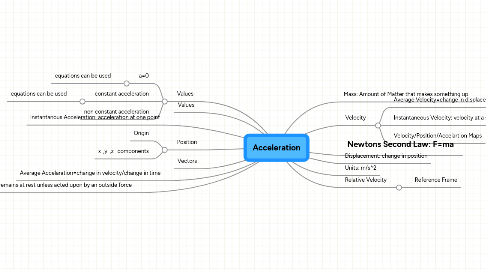# Acceleration

Get Started. It's FreeAcceleration by## 7. Values

### 7.1. a=0

7.1.1. equations can be used

### 7.2. constant acceleration

7.2.1. equations can be used